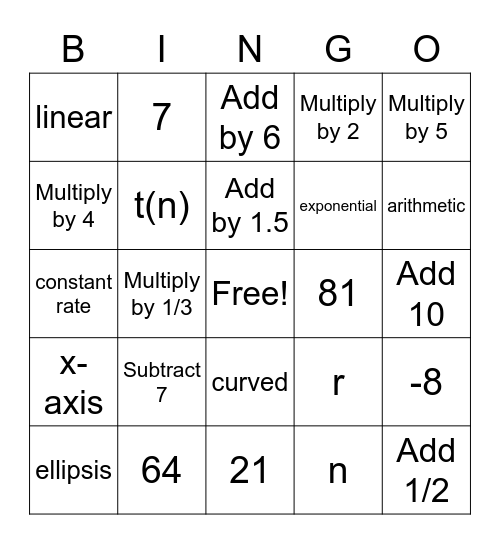# SequencesThis bingo card has a free space and 50 words: Subtract 3, Add 1/2, Multiply by -2, 21, Subtract 7, Multiply by 3, 7, t(1), Multiply by 4, Geometric, t(n), Multiply by 2, -8, r, 36, d, Add by 1.5, 81, t(0), Multiply by 5, Multiply by 1/3, n, linear, exponential, Multiply by -1/2, common ratio, arithmetic, 8, Add by 6, curved, add or subtract, false, 4, Multiply by -4, 64, Subtract 9, discrete, 1, 2, Add 10, multiple or divide, Add 20, 6, common difference, ellipsis, constant rate, increase, decrease, x-axis and y-axis.

## Play Online